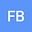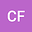••Let $\Omega$ be a bounded domain in $\mathbb{R}^N$ with $N\geqslant 2$, which boundary $\partial\Omega$ is assumed to be a $\mathcal{C}^4$-hypersurface. In this paper we consider the initial-boundary value problem associated with the following thermoelastic plate system $\begin{cases} \partial_t^2u +\Delta^2 u+\Delta\theta=f(u),\ & x\in\Omega,\ t>0, \\ \partial_t\theta-\Delta \theta-\Delta \partial_tu=0,\ & x\in\Omega,\ t>0, \end{cases}$ subject to boundary conditions $\begin{cases} u=\Delta u=0,\ & x\in\partial\Omega,\ t>0,\\ \theta=0,\ & x\in\partial\Omega,\ t>0, \end{cases}$ and initial conditions $u(x,0)=u_0(x),\ \partial_tu(x,0)=v_0(x)\ \mbox{and}\ \theta(x,0)=\theta_0(x),\ x\in\Omega.$ We calculate explicit the fractional powers of the thermoelastic plate operator associated with this system via Balakrishnan integral formula and we present a fractional approximated system. We obtain a result of local well-posedness of the thermoelastic plate system and of its fractional approximations via geometric theory of semilinear parabolic systems.